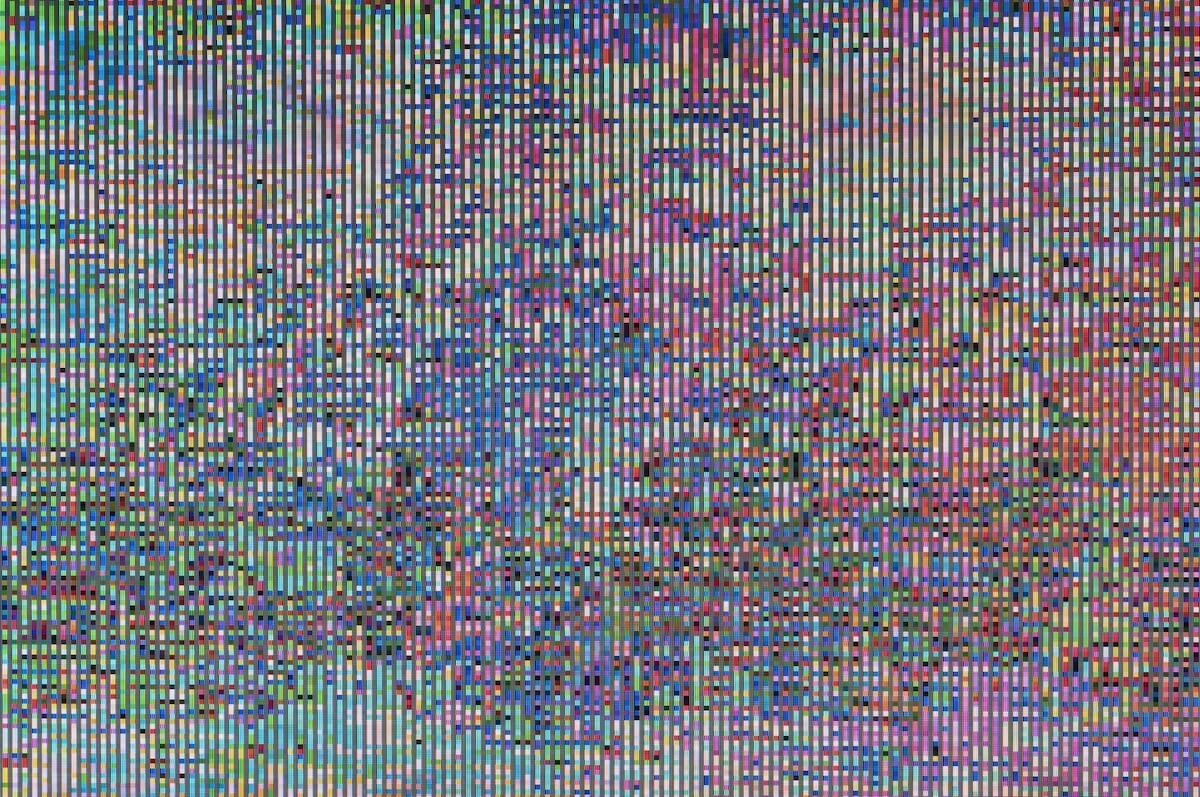# Euclidean distance and noise

## The Euclidean distance measure in use with noisy data

16
.
Apr 2021
by
Michael Welsch
&
Yannik Gaebel

Machine learning very often uses, explicitly or implicitly, the Euclidean distance measure. Let’s take a look at what this means for noisy data and what we can do about it.

A phenomenon occurs at high dimensions that has not yet been observed in 2D and 3D and is often referred to as the curse of dimensions: The distance between the nearest and farthest points approaches the same value, i.e., all points are equally distant from each other, although the information content may differ fundamentally. This phenomenon can be observed for a variety of distance metrics. However, it is particularly pronounced for the Euclidean metric, which in turn works particularly well in 2 and 3 dimensional space. The effect is thus counterintuitive and we must learn to understand it.

Once this effect occurs, it immediately causes the collapse of all machine learning approaches that implicitly or explicitly apply Euclidean or related metrics to high-dimensional data, such as neural networks (gradient methods with corresponding loss definition) or, for example, K-means clustering. Thus, although there would be unique solutions, no solution can be determined if the data is consciously or unconsciously embedded in a Euclidean space.

Let’s take a look at this phenomenon using the following array of curves.

The functional sequences are represented or sampled by an array of 100 numerical values each. Each individual value of these curves is now modulated with a noise generator. Each repetition of this experiment leads to a different expression of the individual values.

Now, if you naively determine the Euclidean distance between two of these noise-modulated curves embedded in a Euclidean space with 100 dimensions, you will determine very large distances or a very large dissimilarity. This is obviously wrong. The output curves are exactly the same and so are the parameters of the noise generator. The information content in both curves is the same. If one were to consider the curves without noise and the noise individually, the distances would be zero with the same offset.

Plotting all pairwise Euclidean distances in a distance matrix as a heat map and successively increasing the noise component, we see how all distances decay and approach a mean distance.

## How can the error be fixed?

With a-priori information about the type of noise (independent on all singular values) and the type of array (a continuous function), even if the data are embedded in a 100dimensional space, an appropriate regularization procedure can be chosen and the noise can be separated again except for numerical artifacts, since the noise occurs 100 times independently. For this purpose, we employ so-called inverse diffusion algorithms, which inversely simulate the emergence of the noise and can therefore “turn down” the noise. We have implemented procedures for this in the Metric Framework, which work not only for simple curves, but for arbitrary data. Regularization based on inverse diffusion is very effective, especially when the noise is rudimentarily normally distributed.

We decompose each data set in this way into its curve without noise and its noise component. The distance is now the Euclidean distance of the regularized curves plus the Euclidean distance between the CDFs of the noise components, also called Cramér-von Mises distance.

Repeating the experiment with this modified metric, we see that the distances decay much less and the structures are largely preserved.

## What does this mean for practice?

Data must be collected with as little noise as possible for machine learning. Alternatively, noise can be removed before training, but this can only work if one has correct a priori information about structural relationships in the data and the nature of the noise. In this example, it was obvious and the ground truth is known. However, this is not the case with field data, especially if the technical circumstances of how the data was collected is not known.

Some algorithms provide an internal regularization. But again, this has to be a random fit to the structure of the data. And that is the trouble with regularization: without appropriate knowledge, it fails and is not a cure-all. There may even be the opposite effect, that an inappropriate regularization (e.g. based on entropy) removes the information content instead of reducing the noise.

This really only leaves the realization for practice: just don’t use noisy data for machine learning unless you know exactly what you are doing and can bring in domain knowledge about the data to suppress the noise.

This is really the swan song to any AutoML approach. It’s the data quality that matters, not the algorithm.

By the way, at PANDA we always record data, whether time series or images, in the highest quality, at least in such a high resolution that remaining noise can be safely removed by oversampling.

We do not only optimize production processes, but also our website! For this, we use tools such as cookies for analysis and marketing purposes. You can change your cookie settings at any time. Information and Settings